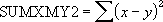## Excel Office

Excel How Tos, Tutorials, Tips & Tricks, Shortcuts

# SUMXMY2 function: Description, Usage, Syntax, Examples and Explanation

## What is SUMXMY2 function in Excel?

SUMXMY2 function is one of the Math and Trig functions in Microsoft Excel that returns the sum of squares of differences of corresponding values in two arrays.

## Syntax of SUMXMY2 function

SUMXMY2(array_x, array_y)

The SUMXMY2 function syntax has the following arguments:

• Array_x: The first array or range of values.
• Array_y:    Required. The second array or range of values.

## SUMXMY2 formula explanation

• The arguments should be either numbers or names, arrays, or references that contain numbers.
• If an array or reference argument contains text, logical values, or empty cells, those values are ignored; however, cells with the value zero are included.
• If array_x and array_y have a different number of values, SUMXMY2 returns the #N/A error value.
• The equation for the sum of squared differences is:## Example of SUMXMY2 function

Steps to follow:

1. Open a new Excel worksheet.

2. Copy data in the following table below and paste it in cell A1

Note: For formulas to show results, select them, press F2 key on your keyboard and then press Enter.

You can adjust the column widths to see all the data, if need be.

 Data First array Second array 2 6 3 5 9 11 1 7 8 5 7 4 5 4 Formula Description (Result) Result =SUMXMY2(A3:A9,B3:B9) Sum of squares of differences of the two arrays above (79) 79 =SUMXMY2({2, 3, 9, 1, 8, 7, 5}, {6, 5, 11, 7, 5, 4, 4}) Sum of squares of differences of the two arrays constants (79) 79